# Position Size Calculator for ThinkorSwim

T

#### Thomas

##### Guest
??? Sorry I don't understand your response.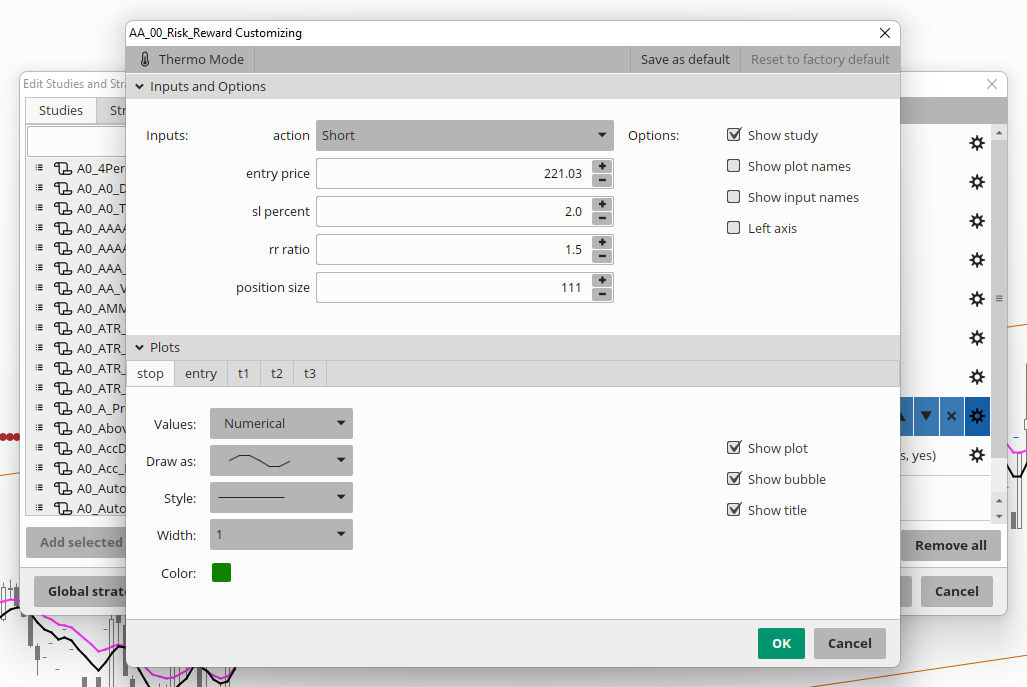You put in the size, entry and stop.

T

#### Thomas

##### GuestYou put in the size, entry and stop.
Or try this label.......
trying to make it as easy as possible http://tos.mx/29GjlCG

Last edited by a moderator:
•Beltrame1

#### mikep

##### New member
Ok thanks Thomas, I'll give it a try.

#### Mr_Wheeler

##### Active member
I came across a script months ago:
but I felt that it was lacking. I just got around to finishing it. I only wish that it could also highlight the price in the active trader.

http://tos.mx/VviLozV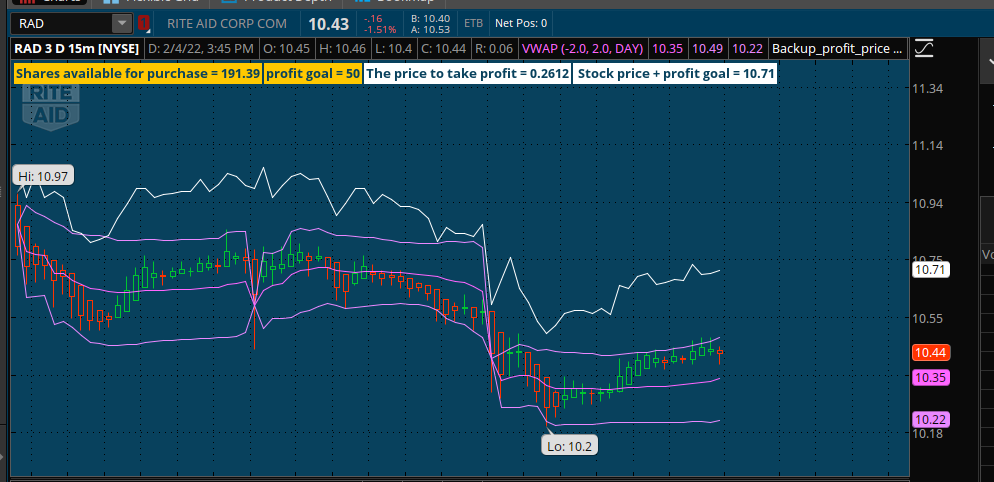Code:
``````# Simple Position Calculator
# Assembled by BenTen at UseThinkScript.com

input Budget = 2000;
def current_price = close;
def Share_Quantity_purchase_limit =  Budget / current_price;

############# labels

AddLabel(yes, Concat("Shares available for purchase = ", Round(Share_Quantity_purchase_limit)), Color.ORANGE);

###############################################################################################

input profit_goal = 50.00;
plot Close_Position = profit_goal/Share_Quantity_purchase_limit ;
Close_Position.SetDefaultColor(GetColor(0));

AddLabel(yes, Concat("profit goal = ", Round(profit_goal)), Color.orange);
AddLabel(yes, Concat("required price change = ", (Close_Position)), Color.white);

def  yellow = profit_goal/Share_Quantity_purchase_limit;
#####################################################################

plot priceLine = current_price + close_Position;;
priceline.SetPaintingStrategy(PaintingStrategy.line);
priceLine.SetLineWeight(1);

AddLabel(yes, Concat(" Stock price + profit goal = ",Round(current_price + close_Position )), Color.white);

#AddLabel(yes, Concat(" The amount of active trader movement  = ",Round(Share_Quantity_purchase_limit + close_Position )), Color.white);``````

Last edited by a moderator:
••Renko_Ranger, Beltrame1, SilverWolf and 1 other person

#### yoojay

##### New member
So, this code is from Pelonsax .
Scenario 3 fits my strategy best so what i'm trying to do to make it even better by automatically input the stop price at the previous 5 min candle closed.
This code is for long, can we do on short side as well?
Thank you
Code:
``````#
# PositionSizingCalculator
#
# Author: RamonDV. AKA Pelonsax
#
#

input Risk_Amount = 100;
input Shares = 1000;
input Stop_Price = 100.00;
input Choose_Size_and_Risk = yes; #can't choose stop
input Choose_Size_and_Stop = yes; #can't choose risk
input Choose_Risk_and_Stop = yes; #can't choose size

def Mark = close(PriceType = PriceType.MARK);
def Size  = Risk_Amount / (Mark - Stop_Price);
def Stop = ((Mark * Shares) - Risk_Amount) / Shares;
def Risk = (mark - Stop_Price) * Shares;

#Display current price
AddLabel(yes, "Current Price: " + AsDollars(Mark), color.gray);

#No defined stop
AddLabel(Choose_Size_and_Risk, "No defined stop: " + Shares + " Shares with " + AsDollars(Risk_Amount) + " risk with Stop Loss at " + AsDollars(stop) + "  .", color.gray);

#No defined risk
AddLabel(Choose_Size_and_Stop, "No Defined risk: " + Shares + " Shares with " + AsDollars(risk) + " risk with Stop Loss at " + AsDollars(Stop_Price) + "  .", color.gray);

#No defined size
AddLabel(Choose_Risk_and_Stop, "No defined size: " + Floor(Round(size)) + " Shares with " + AsDollars(Risk_Amount) + " risk with Stop Loss at " + AsDollars(Stop_Price) + "  .", color.gray);``````

#### Mr_Wheeler

##### Active member
I'm combining a custom 5 band VWAP study with the code that I've already uploaded.

The idea is that it would be all-in-one universal study. If you need the VWAP then you disable the 3 moving average plots & labels if you don't want to use 3 moving averages.

I'm also toying with the idea of adding custom audio alerts that use text-to-speach mp3s. I found the Jim Cramer sound board web page. Maybe I'll figure out how to rip the audio for custom alerts as well.

This is my WIP code so far. I recommend that you add it to a new script with the way it messes up study config window.

Code:
``````input Budget = 2000;
def current_price = close;
def Share_Quantity_purchase_limit =  Budget / current_price;
########### Moving Average Lines ###########
input price = close;
input fastLength = 9;
input medLength = 50;
input slowLength = 200;
input displace = 0;
input averageType = AverageType.WILDERS;

plot fastAvg = MovingAverage(averageType, price[-displace], fastLength);
plot medAvg = MovingAverage(averageType, price[-displace], medLength);
plot slowAvg = MovingAverage(averageType, price[-displace], slowLength);

fastAvg.SetDefaultColor(CreateColor(51, 204, 255));
medAvg.SetDefaultColor(CreateColor(255, 95, 95));
slowAvg.SetDefaultColor(Color.WHITE);

fastAvg.SetLineWeight(2);
medAvg.SetLineWeight(2);
slowAvg.SetLineWeight(2);

fastAvg.SetPaintingStrategy(PaintingStrategy.DASHES);
medAvg.SetPaintingStrategy(PaintingStrategy.DASHES);
slowAvg.SetPaintingStrategy(PaintingStrategy.DASHES);

#####################################################

input BbPeriod      = 9;
input BbDeviations  = 1;
input UseAtrFilter  = yes;
input AtrPeriod     = 5;
input HideArrows    = no;

def BBUpper = SimpleMovingAvg(close, BbPeriod) + StDev(close, BbPeriod) * BbDeviations;
def BBLower = SimpleMovingAvg(close, BbPeriod) - StDev(close, BbPeriod) * BbDeviations;

def BBSignal = if close > BBUpper then 1 else if close < BBLower then -1 else 0;

def TrendLine =
if BBSignal == 1 and UseAtrFilter == 1 then
Max(low - ATR(AtrPeriod), TrendLine)
else if BBSignal == -1 and UseAtrFilter == 1 then
Min(high + ATR(AtrPeriod), TrendLine)
else if BBSignal == 0 and UseAtrFilter == 1 then
TrendLine
else if BBSignal == 1 and UseAtrFilter == 0 then
Max(low, TrendLine)
else if BBSignal == -1 and UseAtrFilter == 0 then
Min(high, TrendLine)
else if BBSignal == 0 and UseAtrFilter == 0 then
TrendLine
else TrendLine;

def iTrend = if TrendLine > TrendLine then 1 else if TrendLine < TrendLine then -1 else iTrend;

plot buy_price = if iTrend == -1 and iTrend == 1 and !HideArrows then TrendLine else Double.NaN;

plot buy_arrow = if iTrend == -1 and iTrend == 1 and !HideArrows then TrendLine else Double.NaN;

plot sell_arrow = if iTrend == 1 and iTrend == -1 and !HideArrows then  TrendLine else Double.NaN;
sell_arrow.SetPaintingStrategy(PaintingStrategy.ARROW_DOWN);
sell_arrow.SetDefaultColor(Color.RED);
sell_arrow.SetLineWeight(5);

plot sell_price = if iTrend == 1 and iTrend == -1 and !HideArrows then  TrendLine else Double.NaN;
sell_price.SetPaintingStrategy(PaintingStrategy.VALUES_BELOW);
sell_price.SetDefaultColor(Color.WHITE);
sell_price.SetLineWeight(3);

########
def arrow_purchase_price = Budget / iTrend ;
######### Label control
input  current_number_of_shares_that_you_can_purchase_at_the_current_price = Yes;
input stock_price_of_the_fast_moving_average_line = Yes;
input stock_price_of_the_Medium_moving_average_line = Yes;
input stock_price_of_the_slow_moving_average_line = Yes;
##########

def greenBuy = if iTrend == -1 and iTrend == 1 and !HideArrows then TrendLine else greenBuy;

AddLabel(current_number_of_shares_that_you_can_purchase_at_the_current_price, Concat("current price shares = ", Round(Share_Quantity_purchase_limit)), Color.ORANGE);

AddLabel(stock_price_of_the_fast_moving_average_line, Concat("MovAvg = ", Round(fastAvg)), (CreateColor(51, 204, 255)));

AddLabel(stock_price_of_the_Medium_moving_average_line, Concat("MovAvg = ", Round(medAvg)), (CreateColor(255, 95, 95)));

AddLabel(Profit_if_you_bought_at_buy_arrow_and_sold_at_the_Med_moving_Avg_price, Concat("Profit @ medAvg = ", Round( Budget / greenBuy  * medAvg - Budget)), (CreateColor(255, 95, 95)));

AddLabel(stock_price_of_the_slow_moving_average_line, Concat("MovAvg = ", Round (slowAvg)), Color.WHITE);

##########################################################################################################################################################
##########################################################################################################################################################
##########################################################################################################################################################
##########################################################################################################################################################
##########################################################################################################################################################

#Four Line VWAP  #Four Line VWAP #Four Line VWAP #Four Line VWAP  #Four Line VWAP #Four Line VWAP #Four Line VWAP  #Four Line VWAP #Four Line VWAP
#Four Line VWAP  #Four Line VWAP #Four Line VWAP #Four Line VWAP  #Four Line VWAP #Four Line VWA #Four Line VWAP  #Four Line VWAP #Four Line VWAPP

##################################################
#SPACER LABEL

# spacer
input Spacer = yes;

addlabel(Spacer, "                                                                                                                                                                                                                                                                                                                                                                                                                                                                                                                                                                                                ",(CreateColor(8,65,93))); # my custom color for my setup.

# end spacer code

##################################################

input Highest_Upper_Band_dev = 2.0;
input Low_Upper_Band_dev = 1.0;

input High_Low_Band_dev = -1.0;
input Lowest_Low_Band_dev = -2.0;

input timeFrame = {default DAY, WEEK, MONTH};

def cap = GetAggregationPeriod();
def errorInAggregation =
timeFrame == timeFrame.DAY and cap >= AggregationPeriod.WEEK or
timeFrame == timeFrame.WEEK and cap >= AggregationPeriod.MONTH;
Assert(!errorInAggregation, "timeFrame should be not less than current chart aggregation period");

def yyyyMmDd = GetYYYYMMDD();
def periodIndx;
switch (timeFrame) {
case DAY:
periodIndx = yyyyMmDd;
case WEEK:
periodIndx = Floor((DaysFromDate(First(yyyyMmDd)) + GetDayOfWeek(First(yyyyMmDd))) / 7);
case MONTH:
periodIndx = RoundDown(yyyyMmDd / 100, 0);
}
def isPeriodRolled = CompoundValue(1, periodIndx != periodIndx, yes);

def volumeSum;
def volumeVwapSum;
def volumeVwap2Sum;

if (isPeriodRolled) {
volumeSum = volume;
volumeVwapSum = volume * vwap;
volumeVwap2Sum = volume * Sqr(vwap);
} else {
volumeSum = CompoundValue(1, volumeSum + volume, volume);
volumeVwapSum = CompoundValue(1, volumeVwapSum + volume * vwap, volume * vwap);
volumeVwap2Sum = CompoundValue(1, volumeVwap2Sum + volume * Sqr(vwap), volume * Sqr(vwap));
}
def Vwap_price = volumeVwapSum / volumeSum;
def deviation = Sqrt(Max(volumeVwap2Sum / volumeSum - Sqr(Vwap_price), 0));

plot VWAP = Vwap_price;
plot Highest_Upper_Band = Vwap_price + Highest_Upper_Band_dev * deviation;
plot Low_Upper_Band =  Vwap_price + Low_Upper_Band_dev * deviation;
plot High_Low_Band =  Vwap_price + High_Low_Band_dev * deviation;
plot Lowest_Low_Band =  Vwap_price + Lowest_Low_Band_dev * deviation;

VWAP.SetDefaultColor(CreateColor(153, 153, 255));
VWAP.SetLineWeight(3);

Highest_Upper_Band.SetDefaultColor(CreateColor(255, 95, 95));
Highest_Upper_Band.SetLineWeight(3);

Low_Upper_Band.SetDefaultColor(GetColor(4));
Low_Upper_Band.SetLineWeight(3);

High_Low_Band.SetDefaultColor(GetColor(4));
High_Low_Band.SetLineWeight(3);

Lowest_Low_Band.SetDefaultColor(CreateColor(255, 95, 95));
Lowest_Low_Band.SetLineWeight(3);

#############################4_band Vwap Control -- inputs#############################

#Disable Upper band cloud
#disable low band cloud

input Current_Upper_Highest_band_price = Yes;
input Profit_if_you_bought_at_current_price_and_sold_at_highest_upper_band = yes;

input Current_Low_upper_band_price = Yes;
input Profit_if_you_bought_at_current_price_and_sold_at_lower_upper_band = yes;

input current_Vwap_price = Yes;
input Profit_if_you_bought_at_current_price_and_sold_at_VWAP = yes;

input current_high_Lower_band_price = yes;
input Profit_if_you_bought_at_current_price_and_sold_at_high_lower_band = yes;

input current_Lowest_band_price = yes;
input Profit_if_you_bought_at_current_price_and_sold_at_lowest_band = yes;

#################################################### Label code.#############################################################

### ### ### highest Upper band ### ### ###

AddLabel(Current_Upper_Highest_band_price, Concat(" highest Upper Band price = ",  Round (Highest_Upper_Band)),(CreateColor(255, 95, 95)));

AddLabel(Profit_if_you_bought_at_current_price_and_sold_at_highest_upper_band, Concat("Current price shares profit @ Highest Upper Band = ", Round( Share_Quantity_purchase_limit  * Highest_Upper_Band - Budget )), (CreateColor(255, 95, 95)));

### ### ### highest Upper band ### ### ###

### ### ### Lower Upper band ### ### ###

AddLabel(Current_Low_upper_band_price, Concat(" Low upper band price = ",  Round (Low_Upper_Band)), Color.orange);

AddLabel(Profit_if_you_bought_at_current_price_and_sold_at_lower_upper_band, Concat("Current price shares profit @ low Upper Band = ", Round( Share_Quantity_purchase_limit  *Low_Upper_Band - Budget )), Color.orange);

### ### ### Lower Upper band ### ### ###

### ### ### VWAP ### ### ###

AddLabel(current_Vwap_price, Concat(" Vwap price = ", Round (VWAP)), Color.viOLET);

AddLabel(Profit_if_you_bought_at_current_price_and_sold_at_high_lower_band, Concat("Current price shares profit @ Vwap = ", Round( Share_Quantity_purchase_limit  * vwap - Budget )), Color.viOLET);

### ### ### VWAP ### ### ###

### ### ### high lower band ### ### ###

AddLabel(current_high_Lower_band_price, Concat(" high low band price = ", Round (High_Low_Band)), Color.orange);

AddLabel(Profit_if_you_bought_at_current_price_and_sold_at_VWAP, Concat("Current price shares profit @ high lower band = ", Round( Share_Quantity_purchase_limit  * High_Low_Band - Budget )), Color.orange);

### ### ### high lower band ### ### ###

### ### ### Lowest lower band ### ### ###

AddLabel(current_Lowest_band_price, Concat(" Lowest band price = ", Round (Lowest_Low_Band)),(CreateColor(255, 95, 95)));

AddLabel(Profit_if_you_bought_at_current_price_and_sold_at_lowest_band, Concat("Current price shares profit @ lowest band = ", Round( Share_Quantity_purchase_limit  * Lowest_Low_Band - Budget )),(CreateColor(255, 95, 95)));

### ### ### Lowest lower band ### ### ###

##########################################################################################################################################################
##########################################################################################################################################################
##########################################################################################################################################################

# spacer
input Spacer2 = yes;

addlabel(Spacer2, "                                                                                                                                                                                                                                                                                                                                                                                                                                                                                                                                                                                                ",(CreateColor(8,65,93))); # my custom color for my setup.

# end spacer code

##########################################################################################################################################################
##########################################################################################################################################################
##########################################################################################################################################################

input Stop_loss_dollar_amount = 250;
input profit_target = 50.0;

input Stop_Loss_targeted_max_loss = yes;
input Stop_Loss_share_price_target = yes;
input The_Current_stock_price_Stop_Loss_share_price_target = yes;

input profit_goal = yes;
input Required_Price_Change_for_profit = yes;
input Stock_Price_Profit_goal_share_price = yes;

AddLabel(Stop_Loss_targeted_max_loss, Concat("Stop Loss targeted max loss = ", Round( Stop_loss_dollar_amount)),Color.white);

AddLabel(Stop_Loss_share_price_target, Concat("Stop Loss share price target = ", Round( Stop_loss_dollar_amount  /  Share_Quantity_purchase_limit )),Color.white);
AddLabel(The_Current_stock_price_Stop_Loss_share_price_target, Concat("The Current stock price minus the Stop Loss share price target share = ", Round( current_price -  (Stop_loss_dollar_amount  /  Share_Quantity_purchase_limit)  )),Color.white);

AddLabel(profit_goal, Concat("profit goal = ", Round( profit_target )), Color.green);
AddLabel(Required_Price_Change_for_profit, Concat("Required Price Change for profit = ", Round( profit_target /  Share_Quantity_purchase_limit )), Color.green);
AddLabel(Stock_Price_Profit_goal_share_price , Concat("Stock Price + Profit goal share price = ", Round( profit_target /  Share_Quantity_purchase_limit + current_price )), Color.green);

plot stop_loss_line = current_price -  (Stop_loss_dollar_amount  /  Share_Quantity_purchase_limit);
plot profit_target_line = profit_target /  Share_Quantity_purchase_limit + current_price;

##########################################################################################################################################################
##########################################################################################################################################################
##########################################################################################################################################################

#def fastAvg_Alert = MovingAverage(averageType, price[-displace], fastLength) crosses MovingAverage(averageType, price[-displace], medLength);

Last edited:
•Beltrame1

#### Mr_Wheeler

##### Active member
I really like this, when a stock is moving fast you don't have the time to figure out your movement and I think the TOS active trader has some issues. Rather than trying to catch the thing when moving fast in either direction I can see the ask or bid price I need. Is there anyway to incorporate a stop loss into this code that allows me to set my max pain.

I am looking for a pain label

Let me try to explain better. The code allows me to set my max risk and gain and tells me what exit price I need to look for. I wish that it had two more fields in the edit that would allow me to set a stop loss percentage and display the stop loss price as the stock is moving. I use the ladder so I can move the stop loss as I see fit or can set a OCO after I'm in the position. Just as trying to quickly figure out profit targets in a rapidly moving stock which this solves I would also like to see stop loss based on my variable which I'm not sure if percentage or dollar would work best. I'm assuming percentage but open to what I may not know or be thinking about. Thank You,

Perhaps I can better explain. Lets assume I set Budget to 3900.00 and set profit goal to 6% and current stock price is \$1.90 Ask
Shares Available = 2052.63
Profit Goal \$234.00
Required Price Change 0.114
Stock Price + Current Goal \$2.01

So the next bit of information I would like to see is where to set stop loss based on a field in setup based on a percentage of Dollar amount so lets say I entered 4% in the setup study filed the screen would display one more displayed field Stop Loss which would be were to set stop loss based on the stock price at the buy less the 4% telling me to set my stop loss in this example at \$1.82

When I'm trying to scalp by the time I figure out the stop loss and set it the trade can be over so the visual would tell me in real time

Do you want a plot line as well?

#### bbheli

##### New member
Do you want a plot line as well?
If not to much trouble that would be nice thank you

# ###################################################################
I am looking for a pain label and a plot line as as well.
When a stock is moving fast you don't have the time to figure out your movement and I think the TOS active trader has some issues. Rather than trying to catch the thing when moving fast in either direction I can see the ask or bid price I need. Is there anyway to incorporate a stop loss into this code that allows me to set my max pain.

Let me try to explain better. The code allows me to set my max risk and gain and tells me what exit price I need to look for. I wish that it had two more fields in the edit that would allow me to set a stop loss percentage and display the stop loss price as the stock is moving. I use the ladder so I can move the stop loss as I see fit or can set a OCO after I'm in the position. Just as trying to quickly figure out profit targets in a rapidly moving stock which this solves I would also like to see stop loss based on my variable which I'm not sure if percentage or dollar would work best. I'm assuming percentage but open to what I may not know or be thinking about. Thank You,

Perhaps I can better explain. Lets assume I set Budget to 3900.00 and set profit goal to 6% and current stock price is \$1.90 Ask
Shares Available = 2052.63
Profit Goal \$234.00
Required Price Change 0.114
Stock Price + Current Goal \$2.01

So the next bit of information I would like to see is where to set stop loss based on a field in setup based on a percentage of Dollar amount so lets say I entered 4% in the setup study filed the screen would display one more displayed field Stop Loss which would be were to set stop loss based on the stock price at the buy less the 4% telling me to set my stop loss in this example at \$1.82

When I'm trying to scalp by the time I figure out the stop loss and set it the trade can be over so the visual would tell me in real time
# #########################################################################

Last edited by a moderator:

#### Mr_Wheeler

##### Active member
If not to much trouble that would be nice thank you

# ###################################################################
I am looking for a pain label and a plot line as as well.
When a stock is moving fast you don't have the time to figure out your movement and I think the TOS active trader has some issues. Rather than trying to catch the thing when moving fast in either direction I can see the ask or bid price I need. Is there anyway to incorporate a stop loss into this code that allows me to set my max pain.

Let me try to explain better. The code allows me to set my max risk and gain and tells me what exit price I need to look for. I wish that it had two more fields in the edit that would allow me to set a stop loss percentage and display the stop loss price as the stock is moving. I use the ladder so I can move the stop loss as I see fit or can set a OCO after I'm in the position. Just as trying to quickly figure out profit targets in a rapidly moving stock which this solves I would also like to see stop loss based on my variable which I'm not sure if percentage or dollar would work best. I'm assuming percentage but open to what I may not know or be thinking about. Thank You,

Perhaps I can better explain. Lets assume I set Budget to 3900.00 and set profit goal to 6% and current stock price is \$1.90 Ask
Shares Available = 2052.63
Profit Goal \$234.00
Required Price Change 0.114
Stock Price + Current Goal \$2.01

So the next bit of information I would like to see is where to set stop loss based on a field in setup based on a percentage of Dollar amount so lets say I entered 4% in the setup study filed the screen would display one more displayed field Stop Loss which would be were to set stop loss based on the stock price at the buy less the 4% telling me to set my stop loss in this example at \$1.82

When I'm trying to scalp by the time I figure out the stop loss and set it the trade can be over so the visual would tell me in real time
# #########################################################################
Here is my work so far.

I edited that previous post with the new code.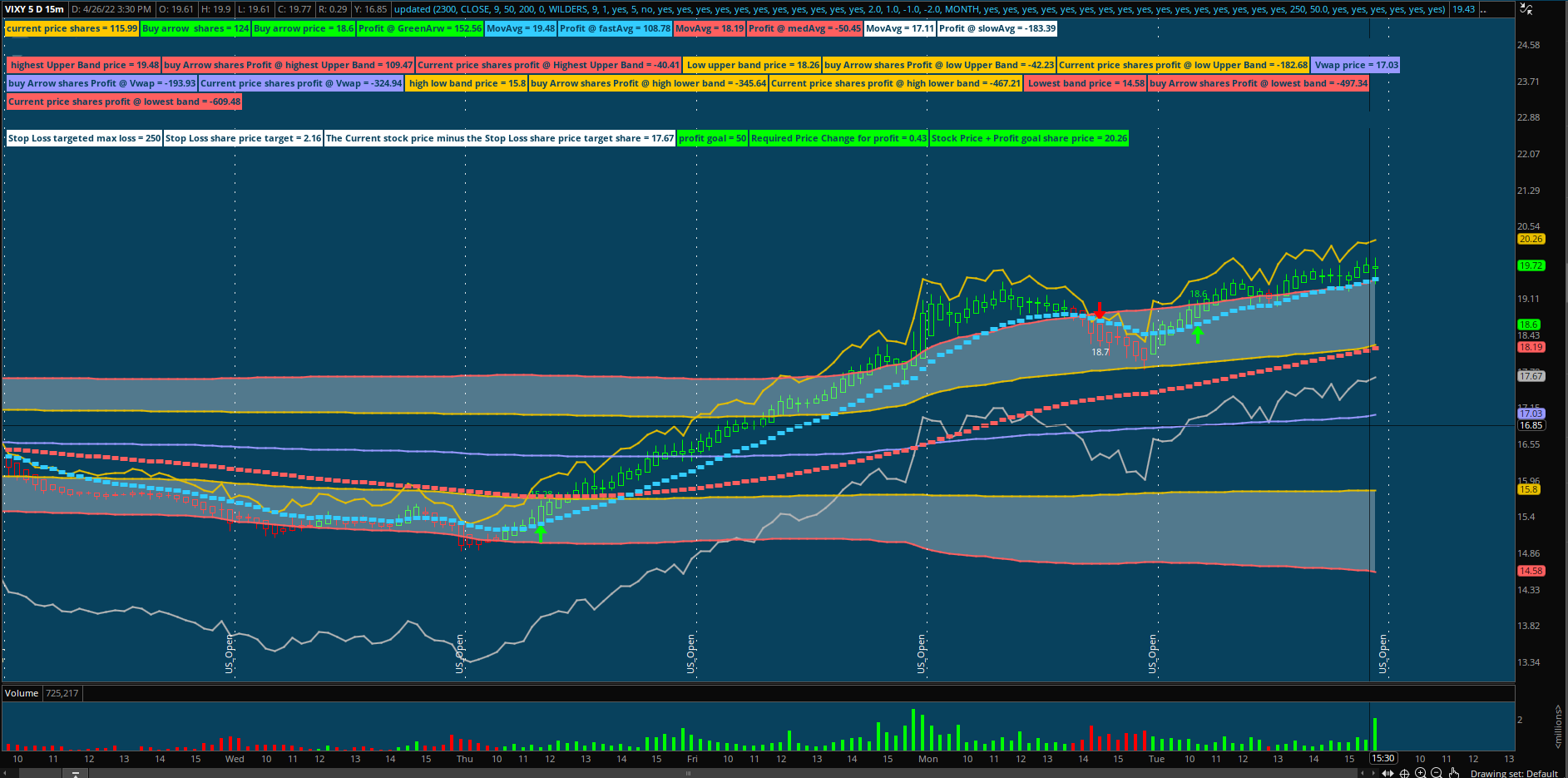Last edited:
••Peace2019 and bbheli

#### Mr_Wheeler

##### Active member
I'll probaly add a label that shows you the percentage of the distance you're away from a moving average cross over.

•Peace2019

#### Peopleg

##### New member
The blue lines is how much the stock would have to move to reach 100 dollars of profit with a shares bought with a purchasing budget of 10,000 dollars.

The 3 white lines indicate the current price & how much a stock would have to increase to make a 1 % move.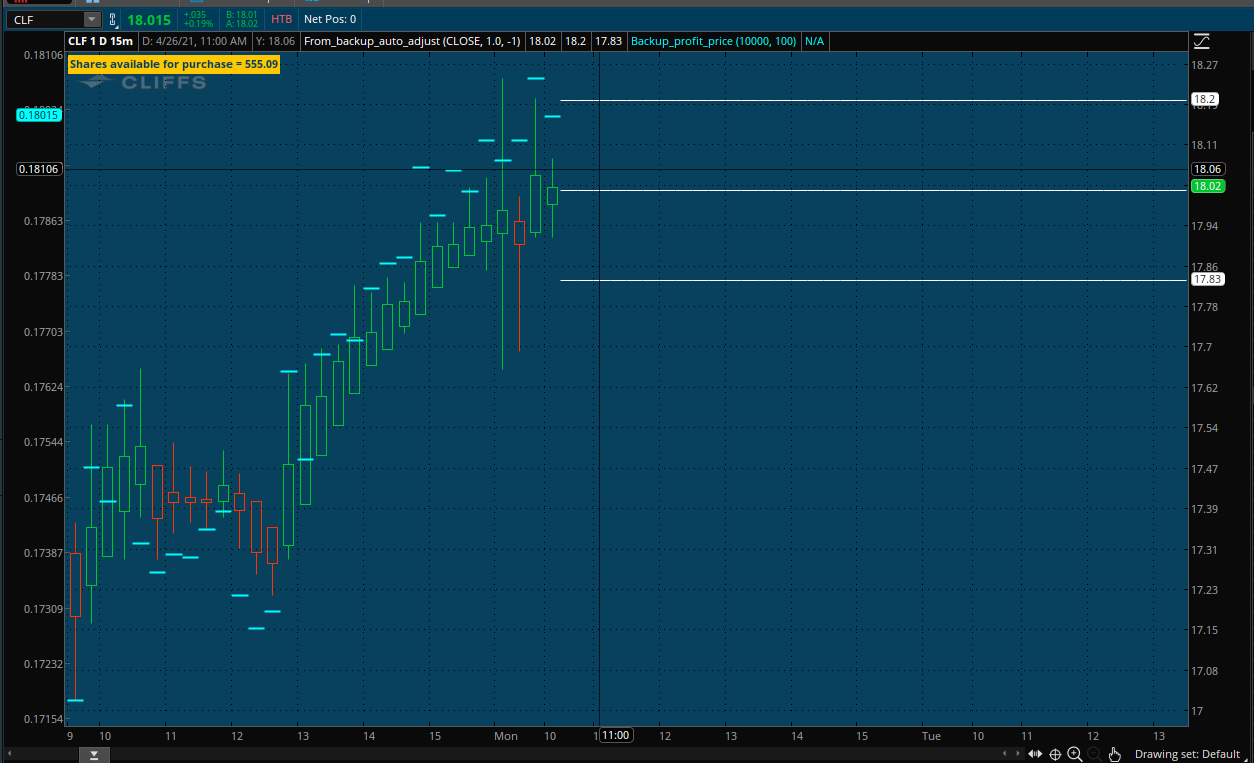Any chance we could get the script for the Blue Lines> THANKS!

#### bbheli

##### New member
Here is my work so far.

I edited that previous post with the new code.I dont see the script for this ? Where can I find it

#### JJJJJJ11111

##### Member
is there anyway to limit the max number of length to like 4 figure on the shares output?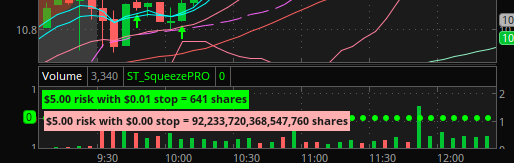Code:
``````#
# PositionSizingCalculator
#
# Author: Kory Gill, @korygill
#
#

input RiskUnit = 5;

def length = 5;

yes,
AsDollars(RiskUnit) + " risk with " + AsDollars(adrrisk) + " stop = " + Floor(Round(RiskUnit/adrrisk)) + " shares",
Color.green
);``````

#### AppleJack1775

##### New member
@CDJay I think this should be able to fulfil your request.

Code:
``````# Simple Position Calculator
# Assembled by BenTen at UseThinkScript.com

input balance = 1000;
def current_price = close;
def limit = balance / current_price;
AddLabel(yes, Concat("Shares available for purchase = ", Round(limit)), color.orange);``````
Thanks for this, very helpful for a long time. Can this be modified so the shares available can be synchronized to the cursor (or something else) in preparation of an approaching target for entry. Right now, I have to calculate the number of shares that I will need when the price is moving toward target. I would rather not have to set/enter limit orders.

•Beltrame1

#### JpMill

##### New member
@GetRichOrDieTrying props to you for having this made. Also, your journey teaches everyone a valuable lesson or two. Thank you for Sharing.
btw, I have such a tool but is excel based and based on 2 different ways of using "R" multiples.
Unfortunately it is proprietary to Theotrade. If I get enough curiosity, I'll ask permission to share it.
The best cal for trading options I saw this post and it was dated 2019 can u let me if if this still valid or is there something better

#### AppleJack1775

##### New member
@CDJay I think this should be able to fulfil your request.

Code:
``````# Simple Position Calculator
# Assembled by BenTen at UseThinkScript.com

input balance = 1000;
def current_price = close;
def limit = balance / current_price;
AddLabel(yes, Concat("Shares available for purchase = ", Round(limit)), color.orange);``````
Thanks BenTen for this, very helpful for a long time...... Can this be modified so the shares available can be synchronized to the cursor or a highlighted bubble in preparation of an approaching target for entry. Right now, I have to calculate the number of shares that I will need when the price is moving toward target/pattern barrier or even back toward the high or low of a previous candle. I would rather not have to set/enter limit orders.

#### QUIKTDR1

##### Active member
VIP
Is there an add-in code that can be added to a strategy to calculate position size by amount of capital attributed to each position?

After strategy signal alerts a buy or sell I 'm looking for an add-on or universal code to add to a strategy that then will determine the position size by amount of capital the trader allots per position automatically with code.

Somehow like:

# Simple Position Calculator
# Assembled by BenTen at UseThinkScript.com

input balance =7500;
def current_price = close;
def limit = balance / current_price;
AddLabel(yes, Concat("Shares available for purchase = ", Round(limit)), color.orange);

only integrated within strategy so when backtesting it shows that amount of shares baased on formua not stagnant 100 shares

#### vollermist

##### New member
Is there an add-in code that can be added to a strategy to calculate position size by amount of capital attributed to each position?

After strategy signal alerts a buy or sell I 'm looking for an add-on or universal code to add to a strategy that then will determine the position size by amount of capital the trader allots per position automatically with code.

Somehow like:

# Simple Position Calculator
# Assembled by BenTen at UseThinkScript.com

input balance =7500;
def current_price = close;
def limit = balance / current_price;
AddLabel(yes, Concat("Shares available for purchase = ", Round(limit)), color.orange);

only integrated within strategy so when backtesting it shows that amount of shares baased on formua not stagnant 100 shares

The following code should be able to be adapted to suit your needs:

Code:
``````#=====================================================
# POSITION SIZING AND RISK
input kellypct = 40.0;
input equity = 5; #hint equity: portfolio equity in thousands
def portfolio = equity * 1000;
input portfolioriskpct = 2.0;
input stoplossriskpct = 1.0;

def tgtentry = close;
def unitrisk = Round(tgtentry * stoplossriskpct / 100,2);
def portfolioriskdollars = Round(portfolio * portfolioriskpct / 100, 0);
def maxtradedollars = Round(portfolio * kellypct / 100, 0);
def kellyshares = maxtradedollars / tgtentry;
def riskshares = portfolioriskdollars / unitrisk;
def shares = GetValue(round(Min(riskshares, kellyshares), 0), -1);
#=====================================================``````

This position Sizer is based on the idea of the Kelly Criterion vs. maximum desired risk (as Kelly is often riskier and can sink you fast if you don't watch out.

Last edited:

### Not the exact question you're looking for?

87k+ Posts
302 Online## The Market Trading Game Changer

Join 2,500+ subscribers inside the useThinkScript VIP Membership Club
• Exclusive indicators
• Proven strategies & setups
• Private Discord community
• Exclusive members-only content
• 1 full year of unlimited support

What is useThinkScript?

useThinkScript is the #1 community of stock market investors using indicators and other tools to power their trading strategies. Traders of all skill levels use our forums to learn about scripting and indicators, help each other, and discover new ways to gain an edge in the markets.

How do I get started?

We get it. Our forum can be intimidating, if not overwhelming. With thousands of topics, tens of thousands of posts, our community has created an incredibly deep knowledge base for stock traders. No one can ever exhaust every resource provided on our site.

If you are new, or just looking for guidance, here are some helpful links to get you started.

What are the benefits of VIP Membership?